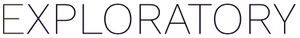# Smart Moving Average by Date and Group

#1

Hi, I am working with a large time-series data set, which is organized by site and day. I have daily temperatures and I want to calculate a consecutive 7 day moving average of these data in a way that recognizes gaps in dates (return NA) and shifts to new sites (should restart calculation). I want the function to return NA values anytime there are data gaps in the 7-day timespan.

It seems like the roll_mean function should work but it doesn’t account for data gaps and shifts in group (sites). Any help is greatly appreciated. I am new to Exploratory and rusty with my R coding skills.

There are lots of time-consuming ways to force this and I have done it manually for years in Excel, but I just know that there should be a smarter way to approach this task and I have LOTS of data to run this through.

Thanks!
Sarah

#2

Hello @Sarah_Kidd1

Is it a problem that cannot be dealt with by the combination of `group_by(site)` and `roll_mean(fill=NA)`?

• recognizes gaps in dates (return NA)
• shifts to new sites (should restart calculation)

※It may not be useful because I do not understand the relationship between the above part of the question content and the table structure (date, temp, site) accurately.

If the `date` itself is missing, restore the `date` and then perform the above calculation.

I hope this will help.

in the Rscript dataframe, sample data was generated by as follows.

``````N <- 10
SITE <- c("A", "B", "C")
DAY <- seq(as.Date("2020-12-01"), as.Date("2020-12-10"), by = "day")
MU <- c(5, 15, 40)

set.seed(1989)
df <- data.frame(
site = rep(SITE, each = N),
date = rep(DAY,  times = length(SITE)),
temp = unlist(purrr::map(.x = MU, .f = function(x){round(rnorm(n = N, mean = x),2)}))
)

df[5,3] <- NA
df[10,3] <- NA
df[22:23,3] <- NA
df
``````
2 Likes
#3

This works perfectly! HURRAY! THANK YOU!1 Like